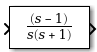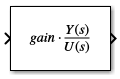# Zero-Pole

Model system using zero-pole-gain transfer function

•Libraries:

## Description

The Zero-Pole block models a system that you define by specifying the zeros, poles, and gain of a Laplace-domain transfer function. You can use this block to model single-input single-output (SISO) and single-input multiple-output (SIMO) systems.

The Zero-Pole block has these requirements:

• The transfer function must have the form

`$H\left(s\right)=K\frac{Z\left(s\right)}{P\left(s\right)}=K\frac{\left(s-Z\left(1\right)\right)\left(s-Z\left(2\right)\right)\dots \left(s-Z\left(m\right)\right)}{\left(s-P\left(1\right)\right)\left(s-P\left(2\right)\right)\dots \left(s-P\left(n\right)\right)},$`

where Z represents the zeros, P represents the poles, and K represents the gain of the transfer function.

• The number of poles must be greater than or equal to the number of zeros.

• Complex poles and zeros must be complex-conjugate pairs.

• For a multiple-output system, all transfer functions must have the same poles. The zeros can differ in value, but the number of zeros for each transfer function must be the same.

Tip

To model a multiple-output system in which the transfer functions have different numbers of zeros or a single zero each, use multiple Zero-Pole blocks.

### Model Single-Output Systems

A single-output system has scalar time-domain input and output signals. To model this type of system:

1. Enter a vector for the zeros of the transfer function in the Zeros field.

2. Enter a vector for the poles of the transfer function in the Poles field.

3. Enter a scalar for the gain of the transfer function in the Gain field.

### Model Multiple-Output Systems

A multiple-output system has a scalar time-domain input signal and a vector time-domain output signal. Each element in the vector output is an output of the system. To model this type of system:

1. Enter a matrix of zeros in the Zeros field.

Each column of the matrix contains the zeros of a transfer function that relates the system input signal to one of the elements in the output signal.

2. Enter a vector for the poles common to all transfer functions of the system in the Poles field.

3. Enter a vector of gains in the Gain field.

Each element is the gain of the corresponding transfer function in the zeros matrix.

## Ports

### Input

expand all

The scalar time-domain input signal for the system.

Data Types: `double`

### Output

expand all

Output of the system modeled as a zero-pole-gain transfer function, provided as a scalar or vector signal.

• When modeling a single-output system, the block produces a scalar time-domain signal.

• When modeling a multiple-output system, the block produces a vector, where each element of the vector is an output of the system.

Data Types: `double`

## Parameters

expand all

Define the matrix of zeros.

• For a single-output system, enter a vector for the zeros of the transfer function.

• For a multiple-output system, enter a matrix. Each column of this matrix contains the zeros for a transfer function that relates the system input to an element of the output vector.

• To model a system without zeros, enter `[]`.

#### Programmatic Use

 Block Parameter: `Zeros` Type: string | character vector Value: vector | matrix Default: `''`

Define the vector of poles.

• For a single-output system, enter a vector for the poles of the transfer function.

• For a multiple-output system, enter a vector for the poles common to all transfer functions of the system.

#### Programmatic Use

 Block Parameter: `Poles` Type: string | character vector Value: vector Default: `'[0 -1]'`

Define the vector of gains.

• For a single-output system, enter a 1-by-1 vector for the gain of the transfer function.

• For a multiple-output system, enter a vector of gains. Each element is the gain of the corresponding transfer function in the zeros matrix.

#### Programmatic Use

 Block Parameter: `Gain` Type: string | character vector Value: vector Default: `''`

Specify whether the zeros, poles, and gains are tunable in accelerator and rapid accelerator simulations and in simulations deployed using Simulink® Compiler™.

• `Auto` — The software determines the level of parameter tunability.

• `Optimized` — The software generates an optimized representation of the zeros, poles, and gain for accelerator, rapid accelerator, and deployed simulations. The zeros, poles, and gain values in the optimized representation are not tunable.

• `Unconstrained` — The values of the Zeros, Poles, and Gain parameters are tunable between accelerator, rapid accelerator, and deployed simulations.

#### Programmatic Use

 Block Parameter: `ParameterTunability` Type: string | character vector Values: `'Auto'` | `'Optimized'` | `'Unconstrained'` Default: `'Auto'`

Variable-step solvers use absolute and relative tolerances when choosing the step size to determine whether the error in state calculations is acceptable.

To inherit the absolute tolerance from the Absolute tolerance configuration parameter, specify this parameter value as `auto` or `-1`.

To specify an absolute tolerance for this block that overrides the value specified for the Absolute tolerance configuration parameter:

• Enter a real, positive scalar value to use to compute all block states.

• Enter a real vector with dimensions that match the dimensions of the continuous states for the block.

#### Programmatic Use

 Block Parameter: ` AbsoluteTolerance` Type: string | character vector Values: `'auto'` | `'-1'` | positive, real scalar | vector of positive, real scalars Default: `'auto'`

Use this parameter to optionally assign names to the states of this block. The names you assign apply only to the states of this block.

• To use default state names, leave this field blank (`''`).

• To assign a single name to a single state, enter the name between quotes. For example, to name a single state `position`, enter `"position"`.

• To assign names to multiple states, specify this parameter value as a cell array of character vectors. Each name in the cell array must be unique. For example, to assign the names `a`, `b`, and `c`, enter `{'a','b','c'}`.

• To specify the names using a MATLAB variable, enter the name of the variable without quotes. For example, to use the variable `names` to specify the state names, enter `names`.

You can specify a number of names that is less than the number of states in the block. In this case, the state names are used for multiple states, and the number of states must divide evenly into the number of state names. For example, when you specify two names for a block that has four states, the first name is used for the first two states, and the second name is used for the last two states.

#### Programmatic Use

 Block Parameter: `ContinuousStateAttributes` Type: string | character vector Values: `' '` | user-defined Default: `' '`

## Block Characteristics

 Data Types `double` Direct Feedthrough `yes` Multidimensional Signals `no` Variable-Size Signals `no` Zero-Crossing Detection `no`

## Tips

The appearance of the transfer function in the Zero-Pole block icon depends on how you specify the zero, pole, and gain parameters.

• If you specify each parameter as an expression or a vector, the block shows the transfer function with the specified zeros, poles, and gain. If you specify a variable in parentheses, the block evaluates the variable.

For example, the image shows how the block looks when you specify the Zeros parameter as `[3 2 1]`, the Poles parameter as `(poles)`, where `poles` is a variable with the value ```[7 5 3 1]```, and the Gain parameter as `gain`.• If you specify each parameter as a variable, the block shows the name of the variable that defines the value for the Gain parameter followed by a generic transfer function.

For example, the image shows how the block looks when you specify the Zeros parameter as the variable `zeros`, the Poles parameter as the variable `poles`, and the Gain parameter as the variable `gain`.• When the block is not large enough to display the transfer function using the parameter values, the transfer function is shown with a numerator of `zeros(s)` and a denominator of `poles(s)`.## Version History

Introduced before R2006a